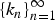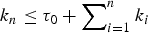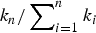Home

# A SPECTRUM OF SERIES–PARALLEL GRAPHS WITH MULTIPLE EDGE EVOLUTION

## Abstract

We discuss a rich family of directed series–parallel (SP) graphs grown by the simultaneous random series or parallel development of multiple edges. The family portrays a spectrum that spans a wide range of SP graphs: from simple models, where only as few as one edge is chosen for evolution at each discrete point in time, to complex hierarchical lattice networks grown by a take-all strategy, where all the edges in the existing network are developed.

The family of SP graphs we discuss is grown from an initial seed graph with τ0 edges under an arbitrary building sequence,$\{k_{n}\}_{n=1}^{\infty}$ , of nonnegative integers (with$k_n \le \tau _0 + \sum\nolimits_{i = 1}^n {k_i}$ , for arbitrary τ0 ≥ 1), that specifies the number of edges subjected to evolution at time n. We study the average north polar degree and show that we can go beyond averages to strong laws. We also find the exact average number of critical edges. The asymptotics of the critical edges are facilitated under the regularity condition that$k_n/\sum\nolimits_{i = 1}^n {k_i}$ converges to a constant (as n → ∞), a natural condition easily met by practical strategies, such as single-edge evolution and take-all choice, and much in between.

Hide All

# A SPECTRUM OF SERIES–PARALLEL GRAPHS WITH MULTIPLE EDGE EVOLUTION

## Metrics

### Full text viewsFull text views reflects the number of PDF downloads, PDFs sent to Google Drive, Dropbox and Kindle and HTML full text views.

Total number of HTML views: 0
Total number of PDF views: 0 *Loading metrics...

### Abstract viewsAbstract views reflect the number of visits to the article landing page.

Total abstract views: 0 *Loading metrics...

* Views captured on Cambridge Core between <date>. This data will be updated every 24 hours.

Usage data cannot currently be displayed.